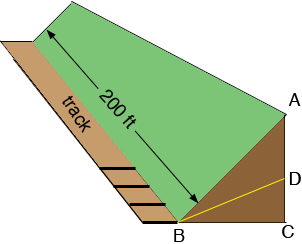SEARCH HOMEMath Central Quandaries & QueriesQuestion from jade, a student: the pennsylvania railroad found it necessary,owing to land slides upon the roadbed,to reduce the angle of inclination of one bank of certain railway cut near pittsburgh,pa.,from an original angle of 45 degrees to a new angle of 30 degrees. the bank as it originally stood was 200 ft.long and had a slant length of 60 ft.. find the amount of the earth removed, if the top level of the bank remained unchanged.In my diagram the length of the line segment AB is 60 ft and the measure of the angle ABC is 45o.Use the sine and cosine of 45o to find the length of the line segments BC and CA and find the area of the triangle ABC. The volume of dirt in the embankment as it exists now is the area of this triangle times the length of the embankment, 200 ft. What is this volume?

The embankment is to be cut down to the line segment BD where the measure of the angle DBC is 30o. Again use trigonometry to find height of the new embankment CD. Use this to calculate the area of the triangle DBC and then the volume of dirt in the new embankment. How much dirt was removed?

PennyMath Central is supported by the University of Regina and The Pacific Institute for the Mathematical Sciences.